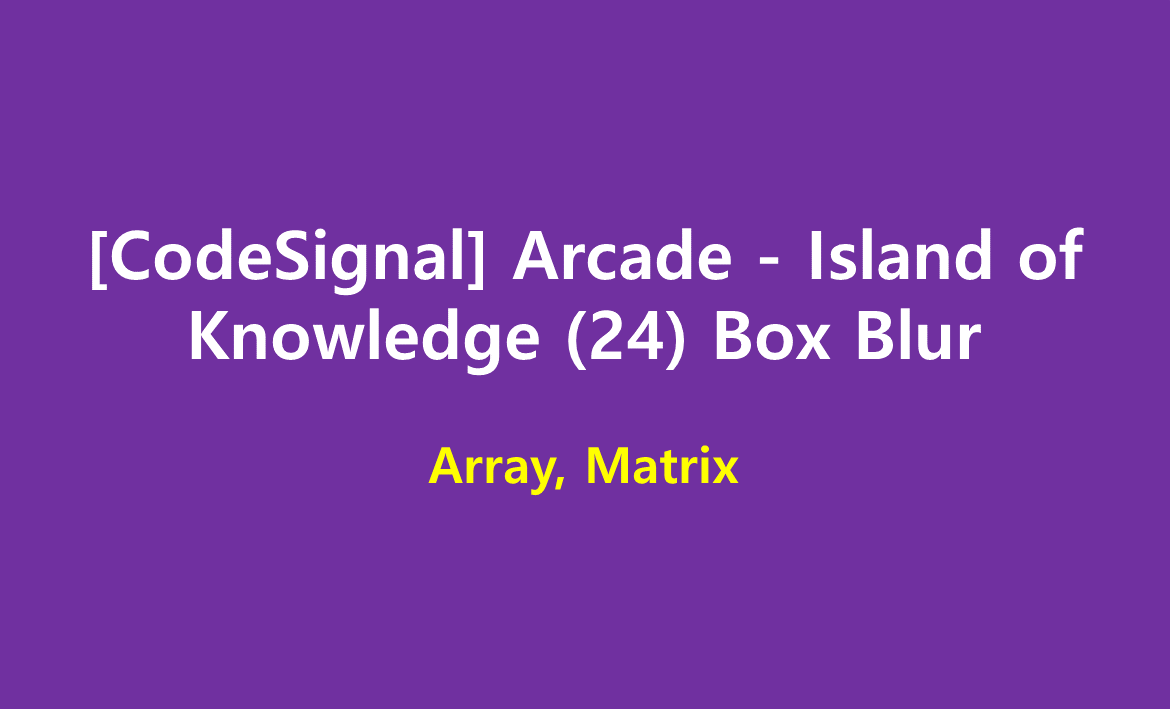# [CodeSignal] Arcade - Island of Knowledge (24) Box Blur

limelimejiwon·2022년 5월 24일
0

## Python으로 공부하는 코딩테스트

목록 보기
65/67## 📄 Description

Last night you partied a little too hard. Now there's a black and white photo of you that's about to go viral! You can't let this ruin your reputation, so you want to apply the box blur algorithm to the photo to hide its content.

The pixels in the input image are represented as integers. The algorithm distorts the input image in the following way: Every pixel x in the output image has a value equal to the average value of the pixel values from the 3 × 3 square that has its center at x, including x itself. All the pixels on the border of x are then removed.

Return the blurred image as an integer, with the fractions rounded down.

### Example

For
image = [[1, 1, 1], [1, 7, 1], [1, 1, 1]]
the output should be solution(image) = [].

To get the value of the middle pixel in the input 3 × 3 square: (1 + 1 + 1 + 1 + 7 + 1 + 1 + 1 + 1) = 15 / 9 = 1.66666 = 1. The border pixels are cropped from the final result.

For
image = [[7, 4, 0, 1], [5, 6, 2, 2], [6, 10, 7, 8], [1, 4, 2, 0]]
the output should be

solution(image) = [[5, 4], [4, 4]]
There are four 3 × 3 squares in the input image, so there should be four integers in the blurred output. To get the first value: (7 + 4 + 0 + 5 + 6 + 2 + 6 + 10 + 7) = 47 / 9 = 5.2222 = 5. The other three integers are obtained the same way, then the surrounding integers are cropped from the final result.

### 📌 Input/Output

• [execution time limit] 4 seconds (py3)

• [input] array.array.integer image

An image, stored as a rectangular matrix of non-negative integers.

### 📌 Guaranteed constraints:

3 ≤ image.length ≤ 100,
3 ≤ image.length ≤ 100,
0 ≤ image[i][j] ≤ 255.

• [output] array.array.integer

A blurred image represented as integers, obtained through the process in the description.

## 💻 My Sumbission

def solution(image):
return answer